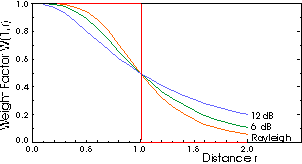### JPL's Wireless Communication Reference Website

#### Chapter: Data Networks Section: Random Access, ALOHA# Vulnerability to Collisions

In an ALOHA network without capture, the probability Q(r) of a successful transmission is
```

Q(r) = exp (-G)

```
where G is total attempted packet transmission traffic on the channel. This does not depend on the distance r between the terminal transmitting the packet and the receiver.

In a radio channel with capture, not all present packets cause harmful interference. So the effective interfering traffic load may be much less than G. Because of propagation path losses, transmissions from nearby terminals will be less vulnerable than packets from remote terminals. We define G(r) as the average number of packets transmitted per slot, per unit of area, from a distance r.

## Vulnerability Circle: a postulated modelAbramson suggested in 1977 to consider interference to be harmful if and only if it arrives from terminals closer to the base station than c r with c a system and propagation constant and r the propagation distance for the test packet.Thus,
```

cr

Q(r) = exp (-  int   G(x)  x dx)

x =0

```
This model ignores channel fading, shadowing and cumulation of interference if multiple packets collide.

## Vulnerability Weight: an Interpretation of exact results

Linnartz showed in 1987 that, considering Rayleigh fading and incoherent cumulation of interference power, there exists a "weight function" W(x,r) such that
```

inf

Q(r) = exp (-  int    W(r,x) G(x)  x dx)

x =0

```
where the weight function is of the form
```

z r^b

W(r,x)   =  -----------------

z r^b   +    x^b

```
where
• r is the distance between the terminal transmitting the test packet and the receiver
• x is a dummy integration variable, which can be interpreted as the distance between interfering terminal and the receiver
• z is the receiver threshold
• b is the propagation path loss law, say 2 < b < 5.
That is, the weight function smooths the all-or-nothing contribution to interference postulated by the vulnerability circle into an analytical function.

If the interfering signals also exhibit shadowing, a weight function also exists.Figure: Weight function W(1,x)

• red: step function: Vulnerability circle
• orange: Vulnerability weight function for Rayleigh fading channel

## Derivation

The derivation of this result uses Laplace image functions of the received signal power.

## Example